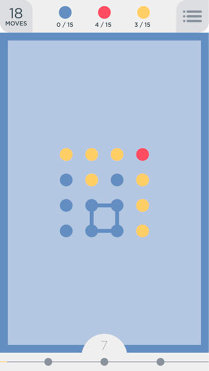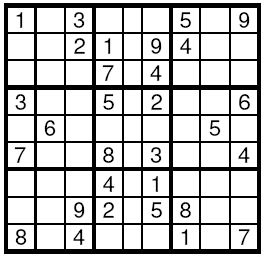# Day7

`未完待续`

## Oil Deposits

`Problem Description`
The GeoSurvComp geologic survey company is responsible for detecting underground oil deposits. GeoSurvComp works with one large rectangular region of land at a time, and creates a grid that divides the land into numerous square plots. It then analyzes each plot separately, using sensing equipment to determine whether or not the plot contains oil. A plot containing oil is called a pocket. If two pockets are adjacent, then they are part of the same oil deposit. Oil deposits can be quite large and may contain numerous pockets. Your job is to determine how many different oil deposits are contained in a grid.
`Input`
The input file contains one or more grids. Each grid begins with a line containing m and n, the number of rows and columns in the grid, separated by a single space. If m = 0 it signals the end of the input; otherwise 1 <= m <= 100 and 1 <= n <= 100. Following this are m lines of n characters each (not counting the end-of-line characters). Each character corresponds to one plot, and is either `*', representing the absence of oil, or`@’, representing an oil pocket.
`Output`
For each grid, output the number of distinct oil deposits. Two different pockets are part of the same oil deposit if they are adjacent horizontally, vertically, or diagonally. An oil deposit will not contain more than 100 pockets.

code:

## How Many Equations Can You Find

`Problem Description`
Now give you an string which only contains 0, 1 ,2 ,3 ,4 ,5 ,6 ,7 ,8 ,9.You are asked to add the sign ‘+’ or ’-’ between the characters. Just like give you a string “12345”, you can work out a string “123+4-5”. Now give you an integer N, please tell me how many ways can you find to make the result of the string equal to N .You can only choose at most one sign between two adjacent characters.
`Input`
Each case contains a string s and a number N . You may be sure the length of the string will not exceed 12 and the absolute value of N will not exceed 999999999999.
`Output`
The output contains one line for each data set : the number of ways you can find to make the equation.

code:

## N皇后问题

`Problem Description`

`Input`

`Output`

code:

## Fox And Two Dots

`Problem Description`
Fox Ciel is playing a mobile puzzle game called “Two Dots”. The basic levels are played on a board of size n × m cells, like this:Each cell contains a dot that has some color. We will use different uppercase Latin characters to express different colors.
The key of this game is to find a cycle that contain dots of same color. Consider 4 blue dots on the picture forming a circle as an example. Formally, we call a sequence of dots d1, d2, …, dk a cycle if and only if it meets the following condition:
These k dots are different: if i ≠ j then di is different from dj.
k is at least 4.
All dots belong to the same color.
For all 1 ≤ i ≤ k - 1: di and di + 1 are adjacent. Also, dk and d1 should also be adjacent. Cells x and y are called adjacent if they share an edge.
Determine if there exists a cycle on the field.
`Input`
The first line contains two integers n and m (2 ≤ n, m ≤ 50): the number of rows and columns of the board.
Then n lines follow, each line contains a string consisting of m characters, expressing colors of dots in each line. Each character is an uppercase Latin letter.
`Output`
Output “Yes” if there exists a cycle, and “No” otherwise.

code:

## 棋盘问题

`Problem Description`

`Input`

`Output`

code:

## Sudoku

`Problem Description`
Sudoku is a very simple task. A square table with 9 rows and 9 columns is divided to 9 smaller squares 3x3 as shown on the Figure. In some of the cells are written decimal digits from 1 to 9. The other cells are empty. The goal is to fill the empty cells with decimal digits from 1 to 9, one digit per cell, in such way that in each row, in each column and in each marked 3x3 subsquare, all the digits from 1 to 9 to appear. Write a program to solve a given Sudoku-task.`Input`
The input data will start with the number of the test cases. For each test case, 9 lines follow, corresponding to the rows of the table. On each line a string of exactly 9 decimal digits is given, corresponding to the cells in this line. If a cell is empty it is represented by 0.
`Output`
For each test case your program should print the solution in the same format as the input data. The empty cells have to be filled according to the rules. If solutions is not unique, then the program may print any one of them.

code:

## 放苹果

`Problem Description`

`Input`

`Output`

code:

## Tempter of the Bone

`Problem Description`

`Input`

“.”: 无障碍点
“X”: 障碍点
“S”: 起点
“D”: 门

`Output`

code:

## Red and Black

`Problem Description`
There is a rectangular room, covered with square tiles. Each tile is colored either red or black. A man is standing on a black tile. From a tile, he can move to one of four adjacent tiles. But he can’t move on red tiles, he can move only on black tiles.

Write a program to count the number of black tiles which he can reach by repeating the moves described above.
`Input`
The input consists of multiple data sets. A data set starts with a line containing two positive integers W and H; W and H are the numbers of tiles in the x- and y- directions, respectively. W and H are not more than 20.

There are H more lines in the data set, each of which includes W characters. Each character represents the color of a tile as follows.

‘.’ - a black tile
‘#’ - a red tile
‘@’ - a man on a black tile(appears exactly once in a data set)
`Output`
For each data set, your program should output a line which contains the number of tiles he can reach from the initial tile (including itself).

code:

---------------- The End ----------------
0%